# Civil Engineering - UPSC Civil Service Exam Questions

46.
The kern of a circular cross-section of radius R is a concentric circular area with a radius of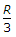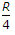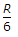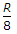Explanation:
No answer description is available. Let's discuss.

47.
On which of the following do the numerical values of Terzaghi's bearing capacity factors depend ?
Angle of internal friction of soil and depth of foundation
Angle of internal friction of soil only
Coefficient of curvature of soil and bulk density of soil
Uniformity coefficient of soil and dry density of soil
Explanation:
No answer description is available. Let's discuss.

48.
A cantilever AB is loaded as shown in the figure. What is the shape of the bending moment diagram for portion AC?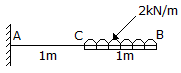Parabolic
Linearly varying with maximum value of the bending moment at C
Linear with constant bending moment value from C to A
Linearly varying with maximum value at A
Explanation:
No answer description is available. Let's discuss.

49.
In the network given above, the critical path of activities is in the given figure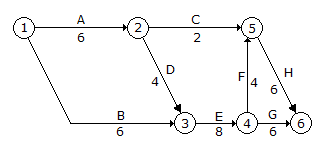A-C-H
B-E-F-H
A-D-E-F-H
A-D-E-G